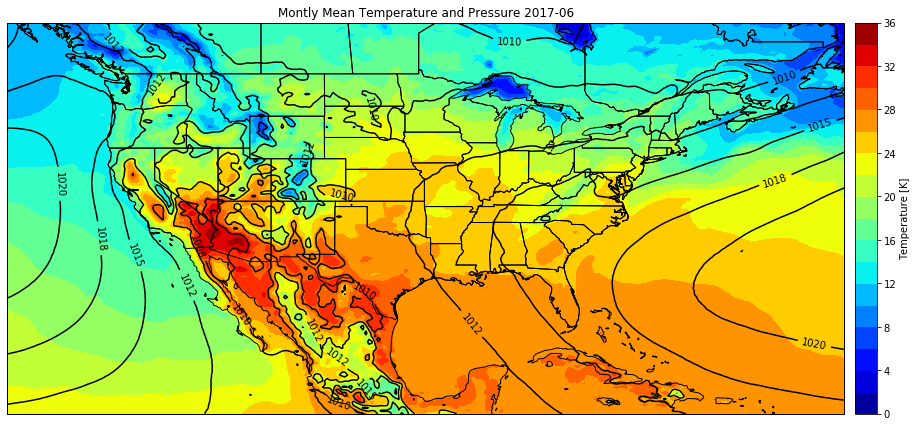# Plotting gridded data onto maps with Cartopy¶

## Needed packages:¶

• xarray
• matplotlib
• numpy
• cartopy
In :
import xarray as xr
import matplotlib.pyplot as plt
import numpy as np
import cartopy.crs as ccrs
import cartopy.feature as cfeature


Data for this demo is a subset of the ERA5 Reanalysis montly-mean, taken from the UCAR/NCAR Research Data Archive.

The data files can be downloaded here. There are two files, one with 2 meter temperature data, and one with MSLP data.

In :
t_data = xr.open_dataset('./409853.VAR_2T.e5.mnth.mean.an.sfc.128_167_2t.regn320sc.2017010100_2017120100.nc')
p_data = xr.open_dataset('./409853.MSL.e5.mnth.mean.an.sfc.128_151_msl.regn320sc.2017010100_2017120100.nc')


Details of these files can be seen by print(t_data) or print(p_data).

Since these variables are in two separate data files, make sure that the two sets of lat/lon data are equal:

In :
print('lat:', np.array_equal(t_data.latitude.values, p_data.latitude.values))
print('lon:', np.array_equal(t_data.longitude.values, p_data.longitude.values))

lat: True
lon: True


Get the data from the files. The temperature and pressure files have a single time dimension, so we can just pull out that single time by taking index [0, :]

In :
temp = t_data.VAR_2T[0,:] - 273.15  # Convert to degC
pres = p_data.MSL[0,:] / 100  # Convert from Pa to hPa
lat = t_data.latitude
lon = t_data.longitude


Now that we have the data, it's time to plot

In :
# Setup the figure
fig = plt.figure(figsize=(15, 15))
ax = plt.subplot(projection=ccrs.PlateCarree())
plt.title(f"Montly Mean Temperature and Pressure 2017-06")

# Plot the data
tplot = ax.contourf(lon, lat, temp, cmap='jet', levels=20)
pplot = ax.contour(lon ,lat, pres, colors='k')

# Cartopy and pyplot colorbars don't play that nice,
# this code manually sets the colorbar axis
posn = ax.get_position()
cbar_ax = fig.add_axes([posn.x0 + posn.width + 0.01, posn.y0, 0.02, posn.height])

plt.colorbar(tplot, cax=cbar_ax, label='Temperature [K]')
plt.clabel(pplot, fmt="%.0f")


<cartopy.mpl.feature_artist.FeatureArtist at 0x7f219e920c90>